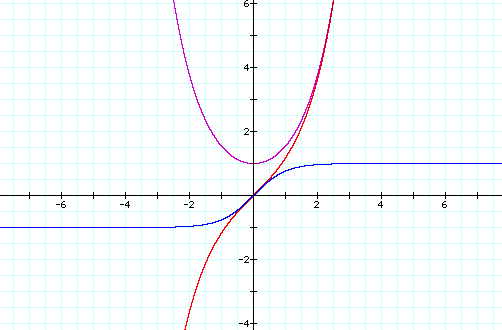#Boost C++ Libraries

...one of the most highly regarded and expertly designed C++ library projects in the world.

### Inverse Hyperbolic Functions Overview

The exponential funtion is defined, for all objects for which this makes sense, as the power series, with `n! = 1x2x3x4x5...xn` (and ```0! = 1``` by definition) being the factorial of `n`. In particular, the exponential function is well defined for real numbers, complex number, quaternions, octonions, and matrices of complex numbers, among others.

Graph of exp on RReal and Imaginary parts of exp on CThe hyperbolic functions are defined as power series which can be computed (for reals, complex, quaternions and octonions) as:

Hyperbolic cosine:Hyperbolic sine:Hyperbolic tangent:Trigonometric functions on R (cos: purple; sin: red; tan: blue)Hyperbolic functions on r (cosh: purple; sinh: red; tanh: blue)The hyperbolic sine is one to one on the set of real numbers, with range the full set of reals, while the hyperbolic tangent is also one to one on the set of real numbers but with range `[0;+∞[`, and therefore both have inverses. The hyperbolic cosine is one to one from `]-∞;+1[` onto `]-∞;-1[` (and from `]+1;+∞[` onto `]-∞;-1[`); the inverse function we use here is defined on `]-∞;-1[` with range `]-∞;+1[`.

The inverse of the hyperbolic tangent is called the Argument hyperbolic tangent, and can be computed as.

The inverse of the hyperbolic sine is called the Argument hyperbolic sine, and can be computed (for `[-1;-1+ε[`) as.

The inverse of the hyperbolic cosine is called the Argument hyperbolic cosine, and can be computed as.

 Copyright © 2006-2010, 2012-2014 Nikhar Agrawal, Anton Bikineev, Paul A. Bristow, Marco Guazzone, Christopher Kormanyos, Hubert Holin, Bruno Lalande, John Maddock, Johan Råde, Gautam Sewani, Benjamin Sobotta, Thijs van den Berg, Daryle Walker and Xiaogang Zhang Distributed under the Boost Software License, Version 1.0. (See accompanying file LICENSE_1_0.txt or copy at http://www.boost.org/LICENSE_1_0.txt)